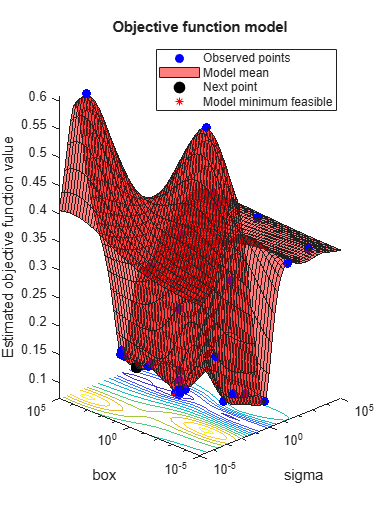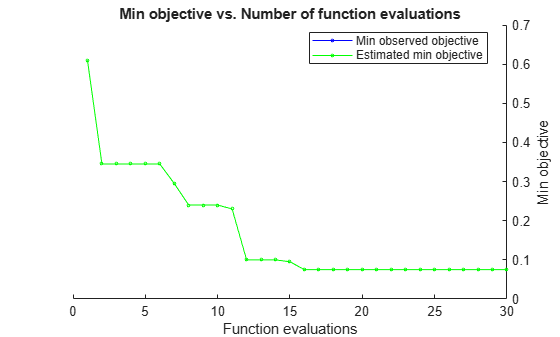Documentation

predictObjectiveEvaluationTime

Predict objective function run times at a set of points

Description

example

time = predictObjectiveEvaluationTime(results,XTable) returns estimated objective evaluation times at the points in XTable.

Examples

collapse all

This example shows how to estimate the objective function evaluation time in an optimized Bayesian model of SVM classification.

Create an optimized SVM model. For details of this model, see Optimize a Cross-Validated SVM Classifier Using bayesopt.

rng default
grnpop = mvnrnd([1,0],eye(2),10);
redpop = mvnrnd([0,1],eye(2),10);
redpts = zeros(100,2);
grnpts = redpts;
for i = 1:100
grnpts(i,:) = mvnrnd(grnpop(randi(10),:),eye(2)*0.02);
redpts(i,:) = mvnrnd(redpop(randi(10),:),eye(2)*0.02);
end
cdata = [grnpts;redpts];
grp = ones(200,1);
grp(101:200) = -1;
c = cvpartition(200,'KFold',10);
sigma = optimizableVariable('sigma',[1e-5,1e5],'Transform','log');
box = optimizableVariable('box',[1e-5,1e5],'Transform','log');
minfn = @(z)kfoldLoss(fitcsvm(cdata,grp,'CVPartition',c,...
'KernelFunction','rbf','BoxConstraint',z.box,...
'KernelScale',z.sigma));
results = bayesopt(minfn,[sigma,box],'IsObjectiveDeterministic',true,...
'AcquisitionFunctionName','expected-improvement-plus','Verbose',0);Predict the evaluation time for various points.

sigma = logspace(-5,5,11)';
box = 1e5*ones(size(sigma));
XTable = table(sigma,box);
time = predictObjectiveEvaluationTime(results,XTable);
[XTable,table(time)]
ans=11×3 table
sigma      box      time
______    _____    _______

1e-05    1e+05    0.24725
0.0001    1e+05    0.22622
0.001    1e+05    0.22658
0.01    1e+05    0.28248
0.1    1e+05    0.38369
1    1e+05    0.39044
10    1e+05      2.097
100    1e+05    0.73441
1000    1e+05    0.15389
10000    1e+05     0.1808
1e+05    1e+05    0.19353

Input Arguments

collapse all

Bayesian optimization results, specified as a BayesianOptimization object.

Prediction points, specified as a table with D columns, where D is the number of variables in the problem. The function performs its predictions on these points.

Data Types: table

Output Arguments

collapse all

Estimated objective evaluation times, returned as an N-by-1 vector, where N is the number of rows of XTable. The estimated values are the means of the posterior distribution of the Gaussian process model of the evaluation times of the objective function.